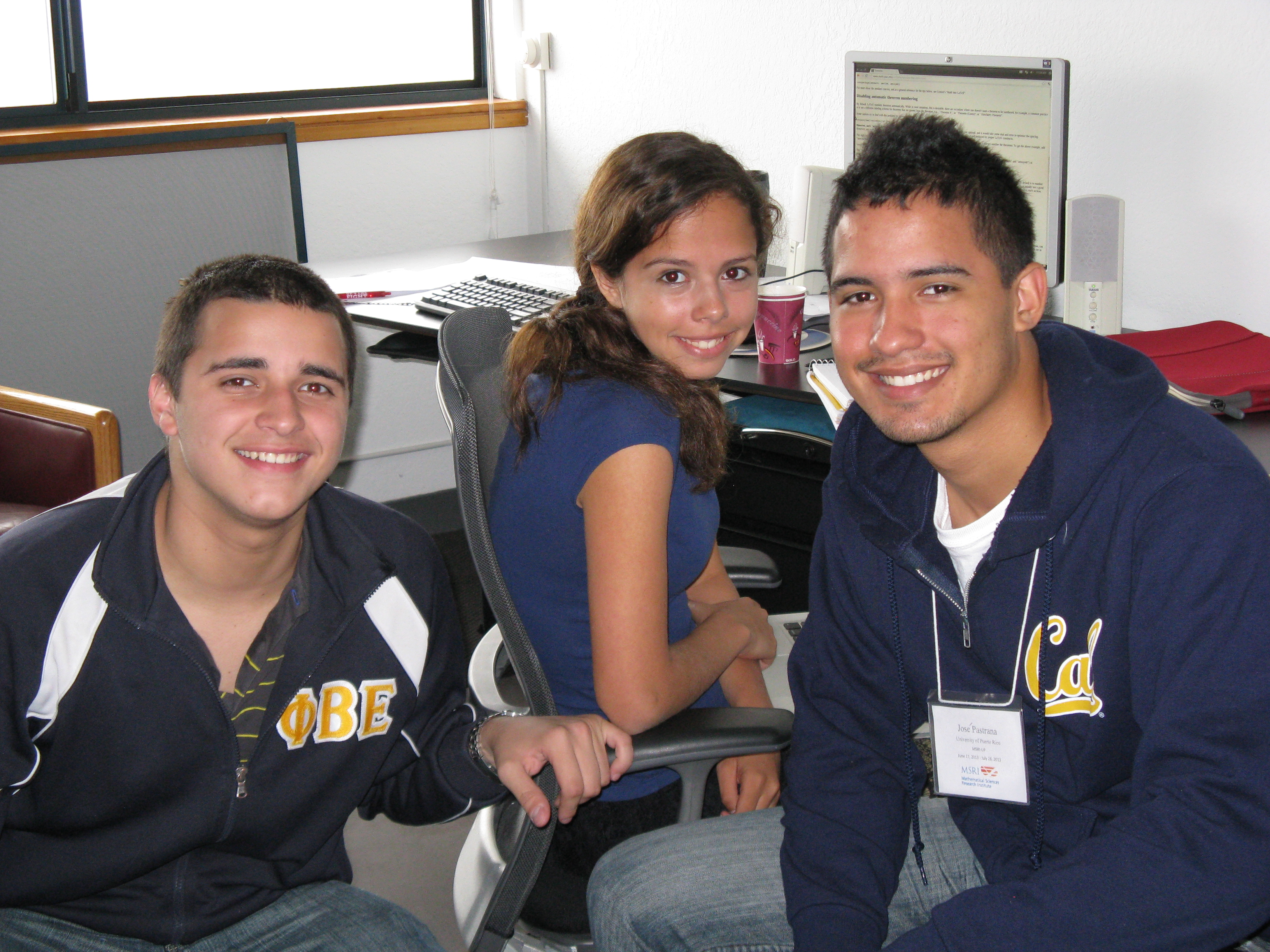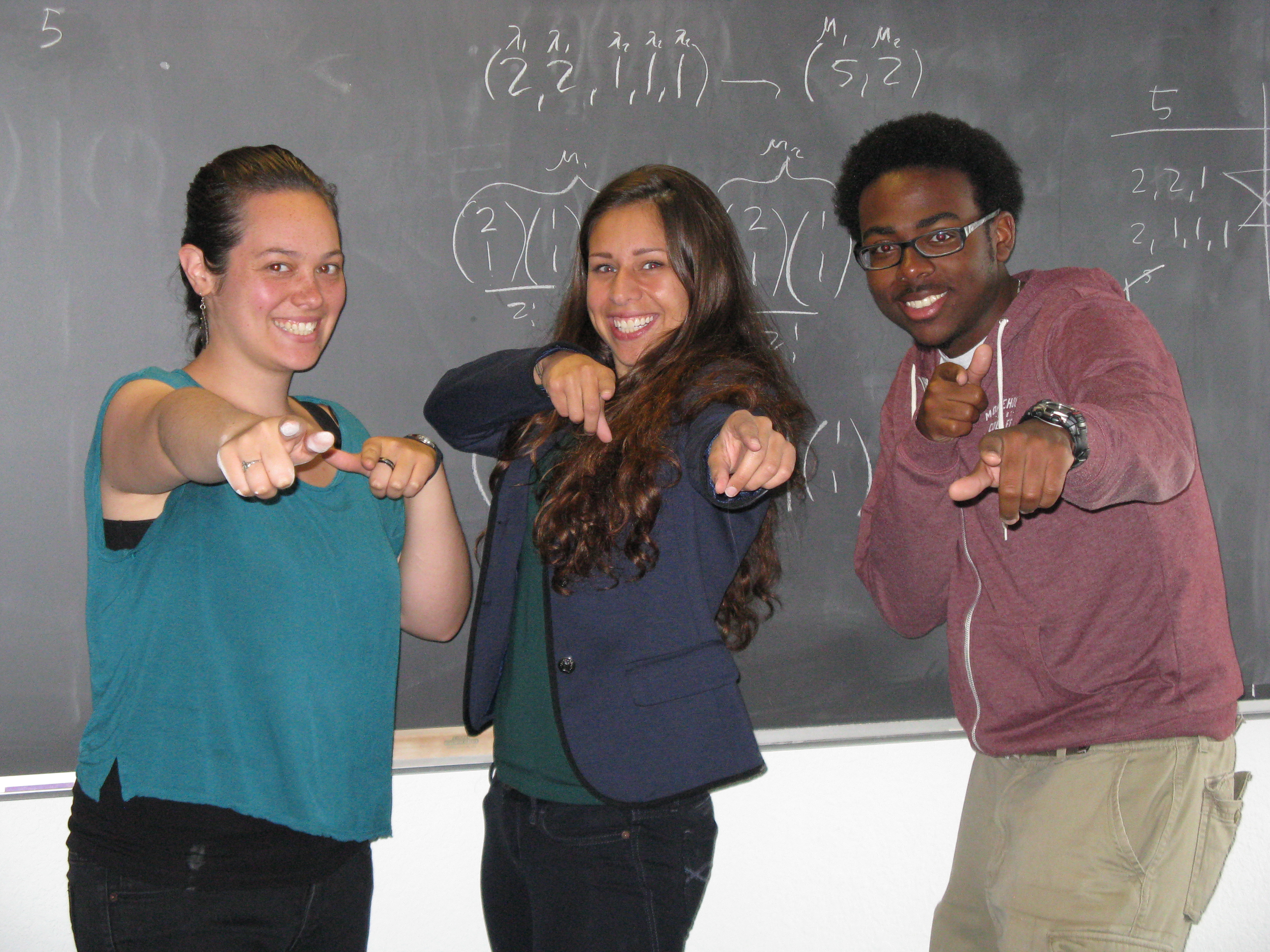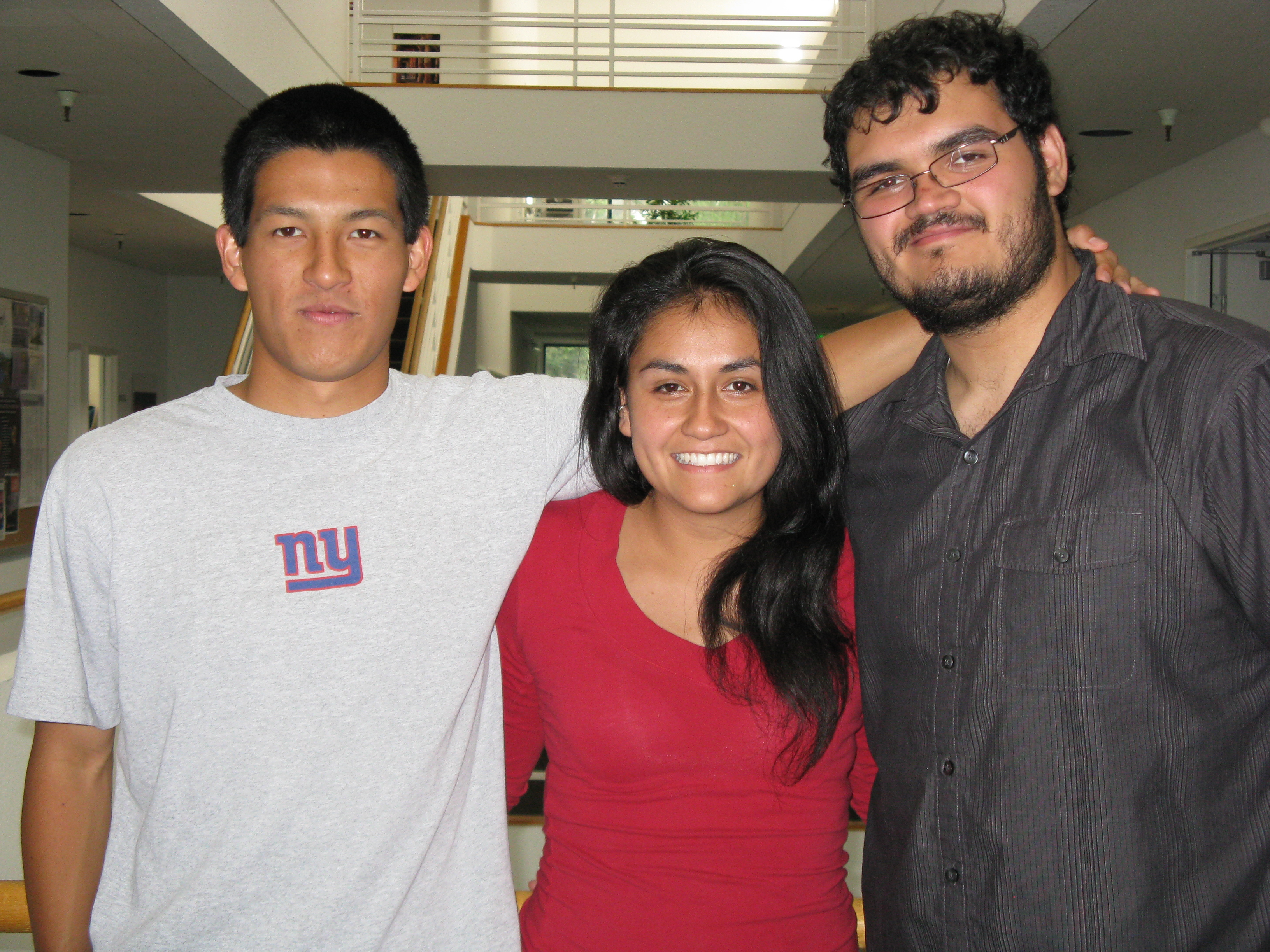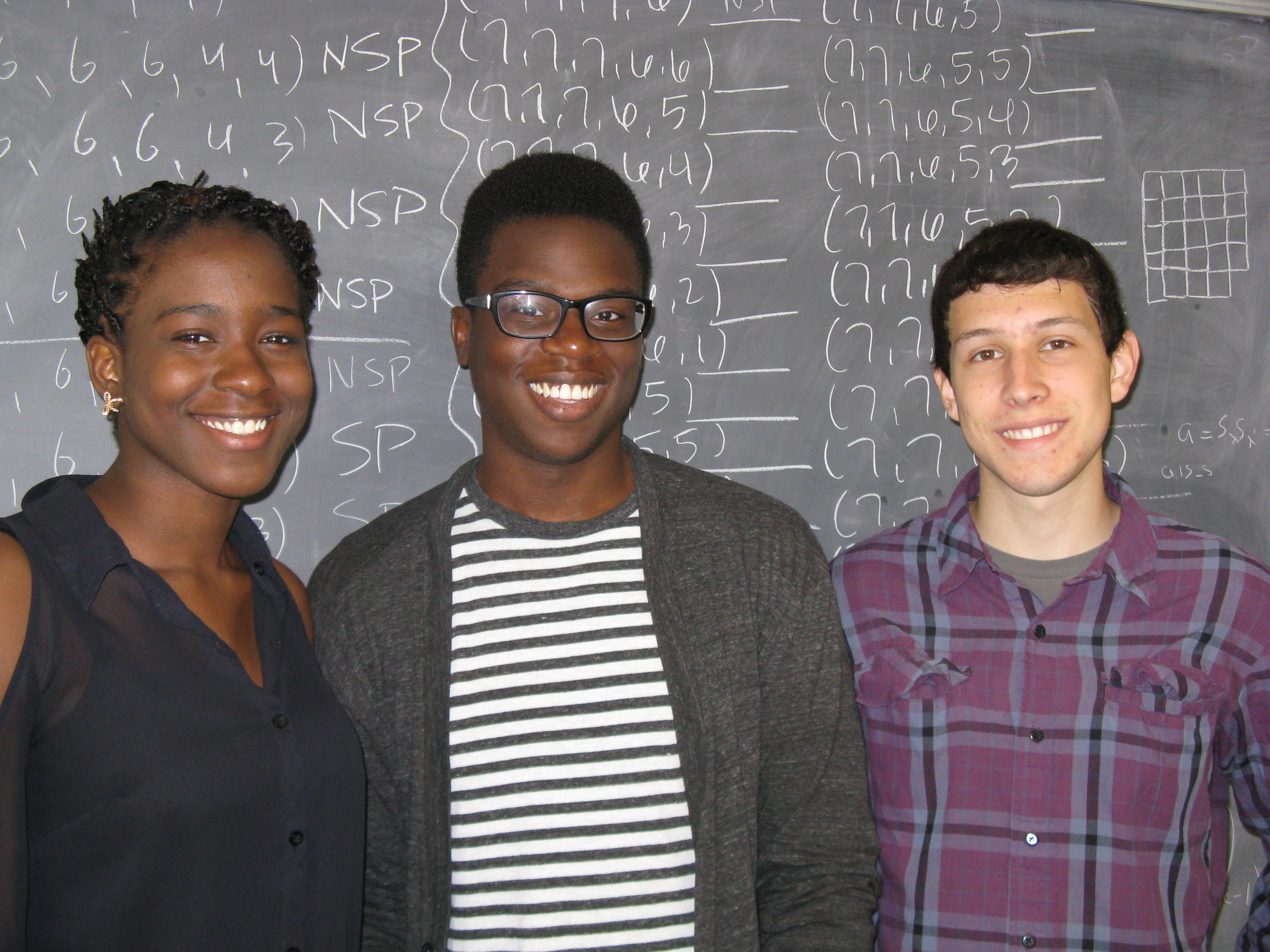/ / / / /

# MSRI-UP 2013: Algebraic Combinatorics

 Home Research Topic People Colloquia Research Projects

1. ### Number of permutations with same peak set for signed permutationsTeam: Francis Castro, Jose Pastrana, Rita ZevallosSupervised by: Alexander Diaz and Rosa Orellana

Let $$B_n$$ be the group of all signed permutations of $$[n]$$. A signed permutation has a peak in a position $$i=2, \ldots, n-1$$ if $$\pi_{i-1} < \pi_i > \pi_{i+1}$$. Let $$P(\pi)$$ be the set of peaks of $$\pi$$, $$P(S,n)$$ be the set of signed permutations $$\pi \in B_n$$ such that $$P(\pi)=S$$, and $$\#P(S,n)$$ be the cardinality of $$P(S,n)$$. We show $$\#P(\emptyset,n)=2^{2n-1}$$ and $$\#P(S,n)=p(n)2^{2n-|S|-1}$$ where $$p(n)$$ is some polynomial. We also consider the case in which we add a zero at the beginning of the permutation to also allow peaks at position $$i=1$$.

2. ### The lattice of set partitions and transition matrices of symmetric functionsTeam: Alexandria Burnley, Aquia Richburg, Simone ThirySupervised by: Rosa Orellana and Candice Price

Block tabloids are a combinatorial object introduced by O. Egecioglu and J. Remmel through which the transition matrices between the bases m,e,p and h of the commuting symmetric functions may be defined. There is an alternative way to describe the transition matrices using symmetric functions in non-commuting variables and the lattice of set partitions. Our goal is to study functions on the lattice of set partitions that arise as entries in the transition matrices. Our research explores the relationship between brick tabloids and functions on the lattice of set partitions. For example, we study $$N_{\lambda}(\mu)$$, the number of set partitions of type $$\mu$$ that are larger than or equal to a set partition of type $$\lambda$$, and $$n_{\lambda}(\mu)$$, the number of set partitions of type $$\mu$$ that are less than or equal to a set partition of type $$\lambda$$.
3. ### Permutation Patterns for Real-Valued FunctionsTeam: Alicia Arrua, Gustavo Melendez-Rios, Lynesia TaylorSupervised by: Samuel Ivy and Rosa Orellana

4. Consider the sequence $$[x, f(x), f(f(x)) = f^2(x), \ldots, f^{n-1}(x)]$$ where $$f$$ is a real-valued function and $$n \geq 2$$. We can associate a permutation to every such sequence by comparing it with $$x_1 < x_2 < ... < x_n$$, where $$x_i = f^{j-1}(x)$$ for some $$j = 1, 2, \ldots , n$$. Permutations that arise from these sequences are called allowed permutations and those that do not are called forbidden permutations.For example, the logistic map, $$f : [0,1] \rightarrow [0, 1]$$ is defined by $$f(x) = rx(1- x)$$ where $$0 \leq r \leq 4$$, for any $$x$$. We focus on enumerating the number of forbidden permutations for the logistic map and other functions, including trigonometric functions. For example, for the $$n = 3$$ case, we have found that the one-line permutation (321) is a forbidden permutation for the function $$\sin(\pi x)$$.

5. ### The Algebra of Set PartitionsTeam: Ryan Contreras, Isabel Corona, Matt SarmientoSupervised by: Alexander Diaz and Rosa Orellana

A set partition of [n] = {1, 2, ...,n} is a collection of non-empty disjoint subsets of [n], called blocks, whose union is [n]. A block permutation of [n] consists of two set partitions A and B of [n] having the same number of blocks,and a bijection f : A --> B. We consider the set BPn = {f : A --> B |f is a block permutation}. The elements in BPn can be visualized as graphs having two rows of n labeled vertices, corresponding to A and B. The connected components of each row are determined by connecting the vertices within each block of A and B. We then connect each block of A to the block of B which it maps to under f. The product g · f of two block permutations f : A --> B and g : C --> D of [n] is obtained by gluing the bottom of a graph representing f to the top of a graph representing g, and connecting each block of A to a block in D. We show that BPn is closed under this operation, and hence is a monoid. We have found a set of generators and seek to find a presentation for BPn. We also describe a Hopf algebra structure on BPn.

6. ### Chromatic Symmetric Functions of Trees and UnicyclesTeam: Damien Gonzales, Arman Green, Caprice StanleySupervised by: Rosa Orellana and Candice Price

Given any simple graph, there is a corresponding symmetric function called the chromatic symmetric function (CSF). Introduced by Richard Stanley in 1995, the CSF of a graph G = (V(G), E(G)) is defined as follows: $X_G = \sum_{\kappa} \prod_{v \in V(G)} x_{\kappa (v)}$ where the sum is over all proper colorings $$\kappa$$ of G. A proper coloring is a labeling of a graph such that no two adjacent vertices have the same label. In Geoffrey Scott's senior thesis, published in 2008, several open problems in graph theory were presented. In our poster, we investigate these open problems and generalize some of his results. Our goal is to find necessary conditions for any two graphs that will ensure that they have the same CSF. We first consider two special types of graphs: trees and unicycles and write a program in SAGE to compare the CSF for any simple graph, and thus, compile a library of graphs with a small number of vertices alongside their CSFs.

7. ### On the Schur Positivity of Differences of Products of Schur FunctionsTeam: Jeremiah Emidih, Nadine Jensen, Jeremy MezaSupervised by: Samuel Ivy and Rosa Orellana

The Schur functions are a basis for the ring of symmetric functions indexed by partitions of nonnegative integers. A symmetric function f is called Schur positive if when expressed as a linear combination of Schur functions $$f=\sum_{\lambda}c_{\lambda}s_{\lambda}$$ each coefficient $$c_{\lambda}$$ is nonnegative. We wish to investigate expressions of the form $$s_{\lambda^c}s_{\lambda}-s_{\mu^c}s_{\mu}$$ where $$\lambda$$ partitions n and $$\mu$$ partitions n-1 and the complements $$\lambda^c, \mu^c$$ are taken over a sufficiently large $$m \times m$$ square. We give a necessary condition that if (1) is Schur positive, then $$\mu$$ is contained in $$\lambda$$. Furthermore, we show how conjugating partitions preserve Schur positivity. Lastly, we incorporate the Littlewood Richardson rule to show that particular classes of $$\lambda$$ of $$\mu$$ are never Schur positive.